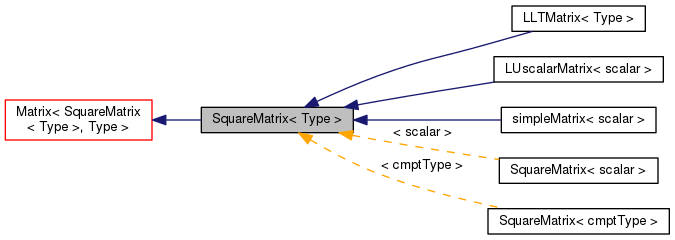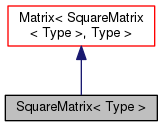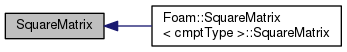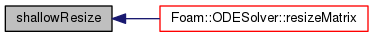The OpenFOAM Foundation
SquareMatrix< Type > Class Template Reference

A templated 2D square matrix of objects of <T>, where the n x n matrix dimension is known and used for subscript bounds checking, etc. More...

Inheritance diagram for SquareMatrix< Type >:[legend]
Collaboration diagram for SquareMatrix< Type >:[legend]

## Public Member Functions

SquareMatrix ()
Null constructor. More...

SquareMatrix (const label n)
Construct given number of rows/columns. More...

template<class MatrixType >
SquareMatrix (const ConstMatrixBlock< MatrixType > &)
Construct from a block of another matrix. More...

template<class MatrixType >
SquareMatrix (const MatrixBlock< MatrixType > &)
Construct from a block of another matrix. More...

SquareMatrix (const label n, const zero)
Construct given number of rows/columns. More...

SquareMatrix (const label m, const label n, const zero)
Construct given number of rows and columns (checked to be equal) More...

SquareMatrix (const label n, const Identity< Type >)
Construct given number of rows/columns. More...

SquareMatrix (const label n, const Type &)
Construct with given number of rows and rows. More...

SquareMatrix (const RectangularMatrix< Type > &)
Construct as copy of a RectangularMatrix. More...

SquareMatrix (Istream &)
Construct from Istream. More...

autoPtr< SquareMatrix< Type > > clone () const
Clone. More...

void setSize (const label m)
Resize the matrix preserving the elements. More...

void shallowResize (const label m)
Resize the matrix without reallocating storage (unsafe) More...

void operator= (const zero)
Assignment of all elements to zero. More...

void operator= (const Identity< Type >)
Assignment elements to the. More...Public Member Functions inherited from Matrix< SquareMatrix< Type >, Type >
Matrix ()
Null constructor. More...

Matrix (const label m, const label n)
Construct given number of rows and columns. More...

Matrix (const label m, const label n, const zero)
Construct with given number of rows and columns. More...

Matrix (const label m, const label n, const Type &)
Construct with given number of rows and columns. More...

Matrix (const mType &)
Copy constructor. More...

Matrix (const Matrix< Form2, Type > &)
Copy constructor from matrix of a different form. More...

Matrix (const ConstMatrixBlock< MatrixType > &)
Construct from a block of another matrix. More...

Matrix (const MatrixBlock< MatrixType > &)
Construct from a block of another matrix. More...

Matrix (Istream &)
Construct from Istream. More...

autoPtr< mTypeclone () const
Clone. More...

~Matrix ()
Destructor. More...

label m () const
Return the number of rows. More...

label n () const
Return the number of columns. More...

label size () const
Return the number of elements in matrix (m*n) More...

const Type * v () const
Return element vector of the constant Matrix. More...

Type * v ()
Return element vector of the Matrix. More...

ConstMatrixBlock< mTypeblock (const label m, const label n, const label mStart, const label nStart) const

ConstMatrixBlock< mTypeblock (const label mStart, const label nStart) const

MatrixBlock< mTypeblock (const label m, const label n, const label mStart, const label nStart)

MatrixBlock< mTypeblock (const label mStart, const label nStart)

Foam::ConstMatrixBlock< Foam::Matrix< SquareMatrix< Type >, Type > > block (const label mStart, const label nStart) const

Foam::MatrixBlock< Foam::Matrix< SquareMatrix< Type >, Type > > block (const label mStart, const label nStart)

ConstMatrixBlock< mTypecol (const label m, const label rowStart) const

ConstMatrixBlock< mTypecol (const label m, const label mStart, const label nStart) const

MatrixBlock< mTypecol (const label m, const label rowStart)

MatrixBlock< mTypecol (const label m, const label mStart, const label nStart)

void checki (const label i) const
Check index i is within valid range (0 ... m-1). More...

void checkj (const label j) const
Check index j is within valid range (0 ... n-1). More...

void clear ()
Clear the Matrix, i.e. set sizes to zero. More...

void transfer (mType &)
Transfer the contents of the argument Matrix into this Matrix. More...

void setSize (const label m, const label n)
Resize the matrix preserving the elements. More...

void shallowResize (const label m, const label n)
Resize the matrix without reallocating storage (unsafe) More...

SquareMatrix< Type > T () const
Return the transpose of the matrix. More...

Type * operator[] (const label)
Return subscript-checked row of Matrix. More...

const Type * operator[] (const label) const
Return subscript-checked row of constant Matrix. More...

const Type & operator() (const label i, const label j) const
(i, j) const element access operator More...

Type & operator() (const label i, const label j)
(i, j) element access operator More...

void operator= (const mType &)
Assignment operator. Takes linear time. More...

void operator= (const ConstMatrixBlock< MatrixType > &)
Assignment to a block of another matrix. More...

void operator= (const MatrixBlock< MatrixType > &)
Assignment to a block of another matrix. More...

void operator= (const zero)
Assignment of all elements to zero. More...

void operator= (const Type &)
Assignment of all elements to the given value. More...Public Types inherited from Matrix< SquareMatrix< Type >, Type >
typedef Matrix< SquareMatrix< Type >, Type > mType
Matrix type. More...

typedef Type cmptType
Component type. More...Static Public Member Functions inherited from Matrix< SquareMatrix< Type >, Type >
static const mTypenull ()
Return a null Matrix. More...

## Detailed Description

### template<class Type> class Foam::SquareMatrix< Type >

A templated 2D square matrix of objects of <T>, where the n x n matrix dimension is known and used for subscript bounds checking, etc.

Source files

Definition at line 58 of file SquareMatrix.H.

## ◆ SquareMatrix() [1/10]

 SquareMatrix ( )
inline

Null constructor.

Definition at line 29 of file SquareMatrixI.H.

Referenced by SquareMatrix< cmptType >::SquareMatrix().

Here is the caller graph for this function:## ◆ SquareMatrix() [2/10]

 SquareMatrix ( const label n )
inline

Construct given number of rows/columns.

Definition at line 36 of file SquareMatrixI.H.

## ◆ SquareMatrix() [3/10]

 SquareMatrix ( const ConstMatrixBlock< MatrixType > & block )
inline

Construct from a block of another matrix.

Definition at line 45 of file SquareMatrixI.H.

## ◆ SquareMatrix() [4/10]

 SquareMatrix ( const MatrixBlock< MatrixType > & block )
inline

Construct from a block of another matrix.

Definition at line 56 of file SquareMatrixI.H.

## ◆ SquareMatrix() [5/10]

 SquareMatrix ( const label n, const zero )
inline

Construct given number of rows/columns.

initializing all elements to zero

Definition at line 66 of file SquareMatrixI.H.

## ◆ SquareMatrix() [6/10]

 SquareMatrix ( const label m, const label n, const zero )
inline

Construct given number of rows and columns (checked to be equal)

initializing all elements to zero

Definition at line 77 of file SquareMatrixI.H.

## ◆ SquareMatrix() [7/10]

 SquareMatrix ( const label n, const Identity< Type > )
inline

Construct given number of rows/columns.

Initializing to the identity matrix

Definition at line 97 of file SquareMatrixI.H.

## ◆ SquareMatrix() [8/10]

 SquareMatrix ( const label n, const Type & t )
inline

Construct with given number of rows and rows.

initializing all elements to the given value

Definition at line 113 of file SquareMatrixI.H.

## ◆ SquareMatrix() [9/10]

 SquareMatrix ( const RectangularMatrix< Type > & RM )
inlineexplicit

Construct as copy of a RectangularMatrix.

which is checked to be square

Definition at line 124 of file SquareMatrixI.H.

## ◆ SquareMatrix() [10/10]

 SquareMatrix ( Istream & is )
inline

Construct from Istream.

Definition at line 141 of file SquareMatrixI.H.

## ◆ clone()

 Foam::autoPtr< Foam::SquareMatrix< Type > > clone ( ) const
inline

Clone.

Definition at line 149 of file SquareMatrixI.H.

## ◆ setSize()

 void setSize ( const label m )
inline

Resize the matrix preserving the elements.

Definition at line 158 of file SquareMatrixI.H.

## ◆ shallowResize()

 void shallowResize ( const label m )
inline

Resize the matrix without reallocating storage (unsafe)

Definition at line 165 of file SquareMatrixI.H.

Referenced by ODESolver::resizeMatrix().

Here is the caller graph for this function:## ◆ operator=() [1/2]

 void operator= ( const zero )

Assignment of all elements to zero.

Definition at line 174 of file SquareMatrixI.H.

## ◆ operator=() [2/2]

 void operator= ( const Identity< Type > )

Assignment elements to the.

Definition at line 181 of file SquareMatrixI.H.

The documentation for this class was generated from the following files: